# Python Geovisualization¶

#### Interactive Jupyter Notebook¶

This notebook provides an introduction to Geovisualization using Python. Both the Matplotlib family and HTML-based visualization are covered. This notebook was created by Becky Vandewalle based off of prior work by Dandong Yin.

## Introduction¶

Visualization is an important technique to be familiar with to visualize geospatial data and analysis results. There are quite a few ways to visualize geospatial data using Python, both using extensions to existing plotting capabilities and through specialized geospatial libraries.

Some useful documentation is listed here:

Official introductory tutorials for Matplotlib https://matplotlib.org/tutorials/index.html#introductory
A relatively-short Matplotlib tutorial http://www.labri.fr/perso/nrougier/teaching/matplotlib/matplotlib.html
Basemap examples: https://matplotlib.org/basemap/users/examples.html

## Setup¶

Run this cell for the rest of the notebook to work!

In :
!pip install --user mapclassify
# aftrer installation, you need to restart kernel

Requirement already satisfied: mapclassify in /opt/conda/lib/python3.7/site-packages (2.1.1)
Requirement already satisfied: pandas in /opt/conda/lib/python3.7/site-packages (from mapclassify) (0.25.3)
Requirement already satisfied: scipy>=0.11 in /opt/conda/lib/python3.7/site-packages (from mapclassify) (1.3.1)
Requirement already satisfied: scikit-learn in /opt/conda/lib/python3.7/site-packages (from mapclassify) (0.21.3)
Requirement already satisfied: deprecated in /opt/conda/lib/python3.7/site-packages (from mapclassify) (1.2.7)
Requirement already satisfied: numpy>=1.3 in /opt/conda/lib/python3.7/site-packages (from mapclassify) (1.17.3)
Requirement already satisfied: pytz>=2017.2 in /opt/conda/lib/python3.7/site-packages (from pandas->mapclassify) (2019.3)
Requirement already satisfied: python-dateutil>=2.6.1 in /opt/conda/lib/python3.7/site-packages (from pandas->mapclassify) (2.8.1)
Requirement already satisfied: joblib>=0.11 in /opt/conda/lib/python3.7/site-packages (from scikit-learn->mapclassify) (0.14.0)
Requirement already satisfied: wrapt<2,>=1.10 in /opt/conda/lib/python3.7/site-packages (from deprecated->mapclassify) (1.11.2)
Requirement already satisfied: six>=1.5 in /opt/conda/lib/python3.7/site-packages (from python-dateutil>=2.6.1->pandas->mapclassify) (1.13.0)

In :
# import required libraries

%matplotlib inline
import os
from datetime import datetime

# set environment variable needed for basemap

os.environ["PROJ_LIB"] = r'/opt/conda/pkgs/proj4-5.2.0-he1b5a44_1006/share/proj/'

import numpy as np
import mpl_toolkits

import pandas as pd
import geopandas as gpd
from geopandas import GeoSeries, GeoDataFrame
from shapely.geometry import Point

#import mapclassify
import matplotlib.pyplot as plt
import matplotlib.patches as patches
from mpl_toolkits.basemap import Basemap

import json
import folium
import mplleaflet


## Matplotlib¶

Matplotlib is a graphic workhorse for Python and is commonly used for graphs and figures. The %matplotlib inline function helps plots to display properly in Jupyter Notebooks.

In :
%matplotlib inline


Plot a simple function. The ; is used to suppress Matplotlib's written output.

In :
# plot 0 - 9

plt.plot(range(10));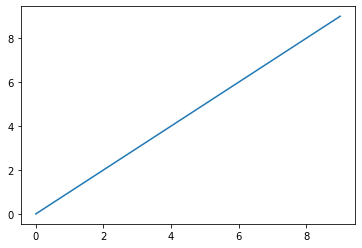### Basic Plot Framework¶

A basic Matplotlib plot contains a Figure, Axes, a Title, and Labels.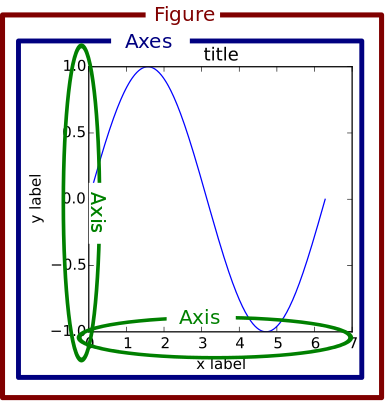It is also possible to include multiple axes in one figure. This image shows three axes.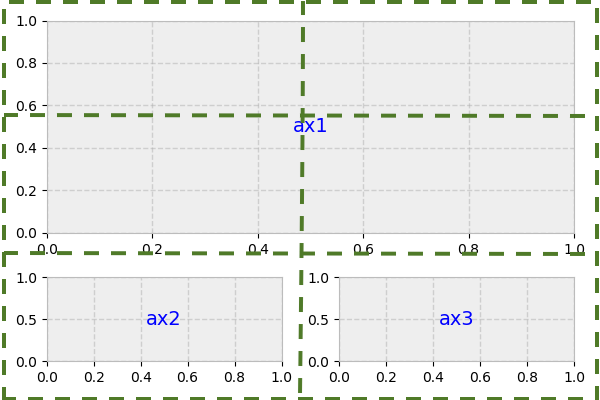### Plotting with Style¶

Using different plotting character keys in the plot function's second argument, you can plot different styles of lines and symbols using different colors.

In the following plot, '+' indicates the symbols should be shaped like crosses, 'r' tells Matplotlib to plot the line in red, and '--' says the line should be dashed.

In :
plt.plot(range(10), '+r--', markersize=10, label='inc');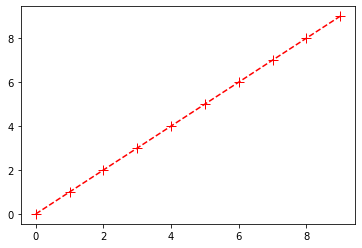Here are a few more examples:

In :
plt.plot(range(10)[::-1], 'b*:', label='dec')
plt.plot([4.5]*10, 'gx-', label='fix')
plt.plot(range(10), 'ko-', label='ver')
plt.legend();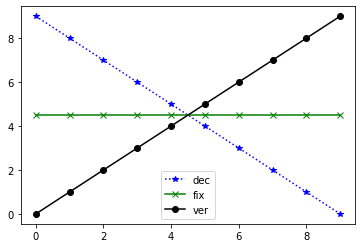### Plotting Shapes¶

You can also draw shapes on a plot using patches.

In :
plt.plot(range(10))
plt.gca().add_patch(patches.Rectangle((0, 0), 9, 9, linewidth=10, edgecolor='cyan', facecolor='none'));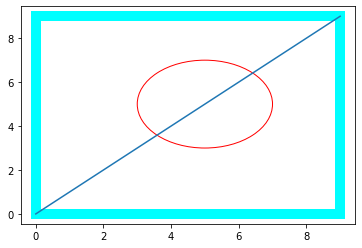A good reference for Matplotlib plotting can be found here.

### Plotting with Numpy¶

Numpy is a Python library that has some useful functions for dealing with number sequences. Below is an example of plotting functions using Matplotlib from a Numpy array.

In :
# evenly sampled time at 200ms intervals
t = np.arange(0., 5., 0.2)

# red dashes, blue squares and green triangles
plt.plot(t, t, 'r--', t, t**2, 'bs', t, t**3, 'g^');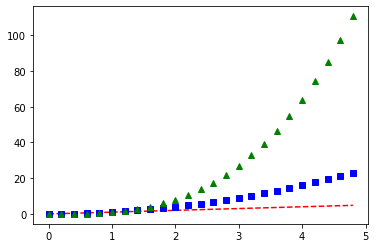Here is an example using random number generation features.

In :
data = {'a': np.arange(50),
'c': np.random.randint(0, 50, 50),
'd': np.random.randn(50)}
data['b'] = data['a'] + 10 * np.random.randn(50)
data['d'] = np.abs(data['d']) * 100

plt.scatter('a', 'b', c='c', s='d', data=data)
plt.xlabel('entry a')
plt.ylabel('entry b');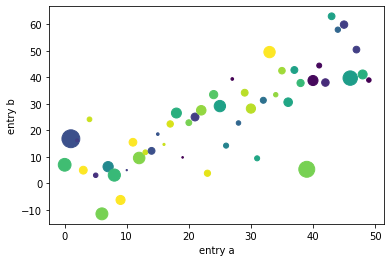### More Plotting Control¶

Knowing the Elements of a Plot can allow you to adjust the plot layout with more fine control. Here are important parts of a plot!Using these we can fine tune labels and line widths for the following histogram.

In :
plt.figure(figsize=(12,10))

mu, sigma = 100, 15
x = mu + sigma * np.random.randn(10000)

n, bins, _ = plt.hist(x, 50, density=1, facecolor='g', alpha=0.75)
plt.xlabel('Value', fontsize=22)
plt.ylabel('Probability', fontsize=22)
plt.title('Histogram', fontsize=22)
plt.tick_params(labelsize=20)
plt.text(60, .025, r'$\mu=100,\ \sigma=15$', fontsize=22)
plt.axis([40, 160, 0, 0.03])
plt.grid(True)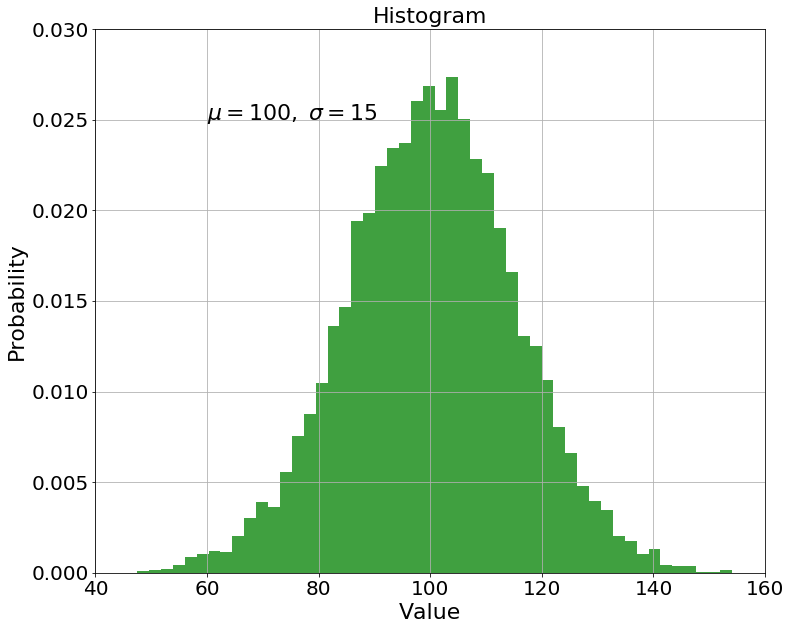## Introducing Basemap¶

Basemap is a library for plotting maps in Python. It handles dealing with coordinate projections, plots user-specified data using Matplotlib, and gathers and clips datasets to draw in the background.

Once you set up a Basemap, you can call different functions, such as the drawcoastlines function to add layers to the map.

The cell below initiates a Basemap by designating projection, resolution, window extent and coordinates.

In :
# set initial values

bmap = Basemap(width=12000000,height=9000000,projection='lcc',
resolution='c',lat_1=45.,lat_2=55,lat_0=50,lon_0=-107.)


Nothing is plotted yet. We can see the type of bmap below.

In :
bmap

Out:
<mpl_toolkits.basemap.Basemap at 0x7fc5e7a18f98>

Now we can start adding to the map:

In :
# draw coastlines

bmap.drawcoastlines();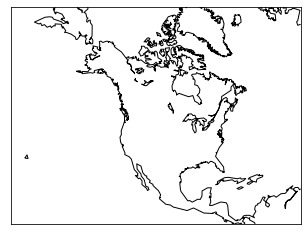By adding a blue background, we can simulate oceans.

In :
# set up map

bmap = Basemap(width=12000000,height=9000000,projection='lcc',
resolution='c',lat_1=45.,lat_2=55,lat_0=50,lon_0=-107.)
bmap.drawcoastlines()

# set map background to blue

bmap.drawmapboundary(fill_color='aqua');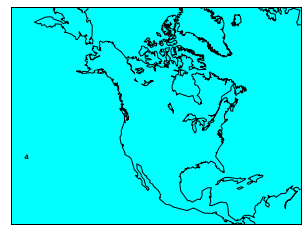Finally we can color the continents so that it looks like they are on top of the ocean.

In :
# set up map

bmap = Basemap(width=12000000,height=9000000,projection='lcc',
resolution='c',lat_1=45.,lat_2=55,lat_0=50,lon_0=-107.)
bmap.drawcoastlines()
bmap.drawmapboundary(fill_color='aqua')

# fill continents, set lake color same as ocean color.

bmap.fillcontinents(color='coral',lake_color='aqua');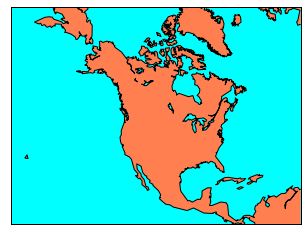### Basemap Backgrounds¶

Several types of preloaded map style options are available in Basemap. Here are a few examples:

Blue Marble:

In :
bmap = Basemap(width=12000000,height=9000000,projection='lcc',
resolution=None,lat_1=45.,lat_2=55,lat_0=50,lon_0=-107.)
bmap.bluemarble();

Clipping input data to the valid range for imshow with RGB data ([0..1] for floats or [0..255] for integers).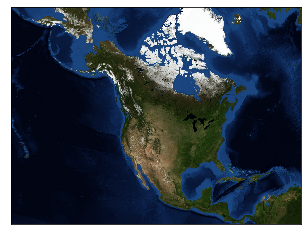In :
bmap = Basemap(width=12000000,height=9000000,projection='lcc',
resolution=None,lat_1=45.,lat_2=55,lat_0=50,lon_0=-107.)

Clipping input data to the valid range for imshow with RGB data ([0..1] for floats or [0..255] for integers).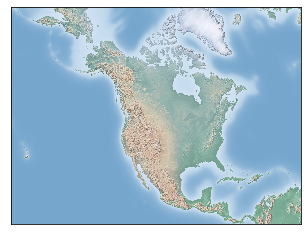ETOPO1 Global Relief Model:

In :
bmap = Basemap(width=12000000,height=9000000,projection='lcc',
resolution=None,lat_1=45.,lat_2=55,lat_0=50,lon_0=-107.)
bmap.etopo();

Clipping input data to the valid range for imshow with RGB data ([0..1] for floats or [0..255] for integers).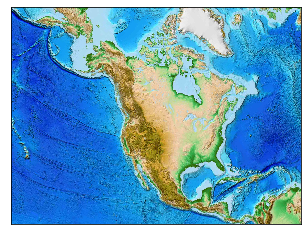### Projecting with Basemap¶

Basemap handles different projections with the projection parameter in the basic basemap. It is fairly easy to draw parallels and meridians with Basemap.

This first map has a Lambert Conformal projection, indicated by lcc.

In :
# setup Lambert Conformal basemap

m = Basemap(width=12000000,height=9000000,projection='lcc',
resolution='c',lat_1=45.,lat_2=55,lat_0=50,lon_0